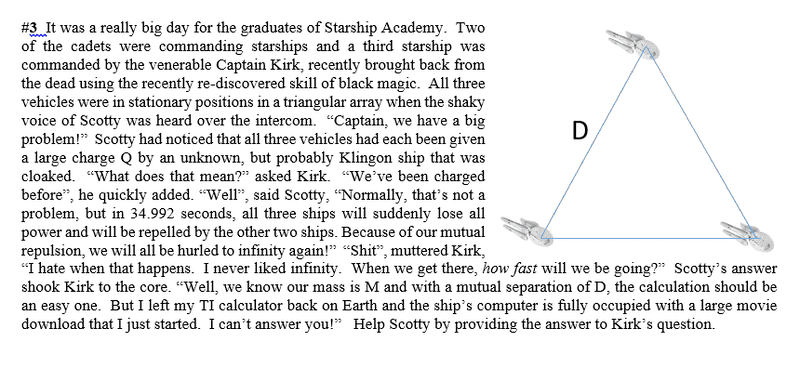# Charge/Field/Velocity Problem

## Homework StatementF=kqQ/D^2=Ma
E=kQ/D^2
D=vt?

## The Attempt at a Solution

So far, I've been able to label the forces on each ship through superposition. But that is all I currently able to understand. I know that my end solution is a speed/velocity, but I am not sure how to get there with the given (Q, t, D, and M)

Any help and direction would be greatly appreciated!

gneill
Mentor
Have you considered a conservation law approach?

I have not because we haven't discussed it. How might I use said approach?

gneill
Mentor
I have not because we haven't discussed it. How might I use said approach?
Usually one considers the trade off between potential and kinetic energy. What form of potential energy is involved here?

Usually one considers the trade off between potential and kinetic energy. What form of potential energy is involved here?

There is definitely potential energy between each ship.

gneill
Mentor
There is definitely potential energy between each ship.
Yes... what type and how much?

Yes... what type and how much?

Electrical Potential Energy
U12=kq1q2/D
U23=kq2q3/D
U13=kq1q3/D

gneill
Mentor
What's the total PE of the system in terms of the variables that you were given?

U(total) = 3* kq^2/D, right?

gneill
Mentor
U(total) = 3* kq^2/D, right?
Looks good.

So how will that change as the ships all tend towards infinite separation? Where will it go?

Looks good.

So how will that change as the ships all tend towards infinite separation? Where will it go?

All the potential will simply turn into kinetic energy right?

gneill
Mentor
All the potential will simply turn into kinetic energy right?
That's right.

That's right.

Alright, so then 3* kq^2/D=1/2Mv^2 and just solve for v

gneill
Mentor
Alright, so then 3* kq^2/D=1/2Mv^2 and just solve for v
Remember that the energy will be split three ways.

Remember that the energy will be split three ways.

So is the multiplying by 3 completely necessary if the charges and D are the same? Couldn't I just solve it as kq^2/D=1/2Mv^2

gneill
Mentor
So is the multiplying by 3 completely necessary if the charges and D are the same? Couldn't I just solve it as kq^2/D=1/2Mv^2
Sure. In your presented solution be sure to state why you are justified in doing it.

Sure. In your presented solution be sure to state why you are justified in doing it.

Ah okay, I understand now! Thank you very much! :)